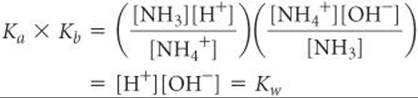﻿ ﻿RELATIONSHIP BETWEEN Ka AND Kb - ACID–BASE EQUILIBRIA - CHEMISTRY THE CENTRAL SCIENCE

## 16 ACID–BASE EQUILIBRIA

### 16.8 RELATIONSHIP BETWEEN Ka AND Kb

We have seen in a qualitative way that the stronger acids have the weaker conjugate bases. To see if we can find a corresponding quantitative relationship, let's consider the NH4+ and NH3 conjugate acid–base pair. Each species reacts with water. For the acid, NH4+ the reaction is:or written in its simpler form:and for the base NH3:and each equilibrium is expressed by a dissociation constant:When we add Equations 16.38 and 16.39, the NH4+ and NH3 species cancel and we are left with the autoionization of water:Recall that when two equations are added to give a third, the equilibrium constant associated with the third equation equals the product of the equilibrium constants of the first two equations.(Section 15.3)

Applying this rule to our present example, when we multiply Ka and Kb, we obtainThus, the product of Ka and Kb is the ion-product constant for water, Kw (Equation 16.16). We expect this result because adding Equations 16.38 and 16.39 gave us the autoionization equilibrium for water, for which the equilibrium constant is Kw.

This relationship is so important that it should receive special attention: The product of the acid-dissociation constant for an acid and the base-dissociation constant for its conjugate base equals the ion-product constant for water:As the strength of an acid increases (Ka gets larger), the strength of its conjugate base must decrease (Kb gets smaller) so that the product Ka × Kb remains 1.0 × 10–14 at 25 °C.TABLE 16.5 demonstrates this relationship. Remember, this important relationship applies only to conjugate acid–base pairs.

By using Equation 16.40, we can calculate Kb for any weak base if we know Ka for its conjugate acid. Similarly, we can calculate Ka for a weak acid if we know Kb for its conjugate base. As a practical consequence, ionization constants are often listed for only one member of a conjugate acid–base pair. For example, Appendix D does not contain Kb values for the anions of weak acids because they can be readily calculated from the tabulated Ka values for their conjugate acids.

TABLE 16.5 • Some Conjugate Acid–Base PairsIf you look up the values for acid-or base-dissociation constants in a chemistry handbook, you may find them expressed as pKa or pKb (that is, –log Ka or –log Kb) (Section 16.4). Equation 16.40 can be written in terms of pKa and pKb by taking the negative logarithm of both sides:GIVE IT SOME THOUGHT

What is the pKa value for HF? What is the pKb value for F?CHEMISTRY PUT TO WORK
Amines and Amine Hydrochlorides

Many low-molecular-weight amines have a fishy odor. Amines and NH3 are produced by the anaerobic (absence of O2) decomposition of dead animal or plant matter. Two such amines with very disagreeable odors are H2N(CH2)4NH2, putrescine, and H2N(CH2)5NH2, cadaverine.

Many drugs, including quinine, codeine, caffeine, and amphetamine, are amines. Like other amines, these substances are weak bases; the amine nitrogen is readily protonated upon treatment with an acid. The resulting products are called acid salts. If we use A as the abbreviation for an amine, the acid salt formed by reaction with hydrochloric acid can be written AH+Cl. It can also be written as A·HCl and referred to as a hydrochloride. Amphetamine hydrochloride, for example, is the acid salt formed by treating amphetamine with HCl:Acid salts are much less volatile, more stable, and generally more water soluble than the corresponding amines. For this reason, many drugs that are amines are sold and administered as acid salts. Some examples of over-the-counter medications that contain amine hydrochlorides as active ingredients are shown inFIGURE 16.14.

RELATED EXERCISES: 16.75, 16.76, 16.104, 16.113, and 16.122FIGURE 16.14 Some over-the-counter medications in which an amine hydrochloride is a major active ingredient.

SAMPLE EXERCISE 16.17 Calculating Ka or Kb for a Conjugate Acid–Base Pair

Calculate (a) Kb for the fluoride ion, (b) Ka for the ammonium ion.

SOLUTION

Analyze We are asked to determine dissociation constants for F, the conjugate base of HF, and NH4+, the conjugate acid of NH3.

Plan We can use the tabulated K values for HF and NH3 and the relationship between Ka and Kb to calculate the ionization constants for their conjugates, F and NH4+.

Solve

(a) For the weak acid HF, Table 16.2 and Appendix D give Ka = 6.8 × 10–4. We can use Equation 16.40 to calculate Kb for the conjugate base, F:(b) For NH3, Table 16.4 and in Appendix D give Kb = 1.8 × 10–5, and this value in Equation 16.40 gives us Ka for the conjugate acid, NH4+:Check The respective K values for Fand NH4+ are listed in Table 16.5, where we see that the values calculated here agree with those in Table 16.5.

PRACTICE EXERCISE

(a) Which of these anions has the largest base-dissociation constant: NO2, PO43–, or N3?

(b) The base quinoline has the structureIts conjugate acid is listed in handbooks as having a pKa of 4.90. What is the base-dissociation constant for quinoline?

Answers: (a) PO43–(Kb = 2.4 × 10–2), (b) Kb = 7.9 × 10–10

﻿